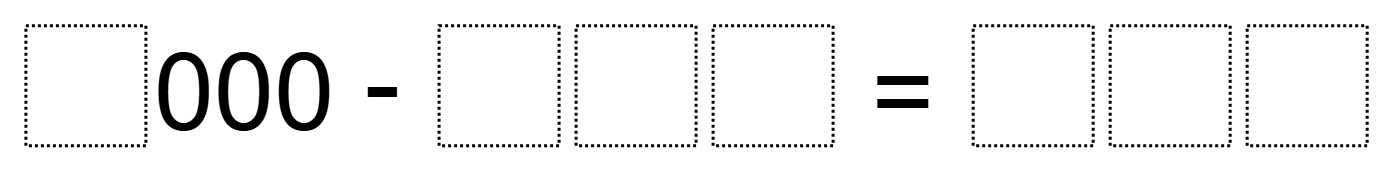# Subtraction with Zeros

Directions: Use the numbers 1-9 at most once each to make this a true subtraction equation.
What is the greatest difference? What is the least difference?### Hint

What number(s) could or could not be be in the first box?
If you found one way, are there other ways that also work?

1000 – 342 = 658 is the greatest difference
1000 – 658 = 342 is the least difference

Source: Ellen Metzger

## Comparing Fractions

Directions: Using the numbers 1-9, each one time only, fill in the blanks to make …

1.Help! Can someone please explain how to solve this problem. We are struggling to not use two nines. I know I have to be missing something but can’t figure it out!
THANKS!

•I start figuring number with the thousands first, then hundreds and so on. I considered if I would need columns to regroup. Got I right away to exactly 10,000
3749 + 6251.

2.We found 1,000-876 = 124

•You have a one in the subtraction and a one in the answer. :/

3.Ms. Foley, you can’t have two ones in it

4.